Electromagnetic Wave Theory for Calculation of Exact Magnetic Field in Case of BWO | OMICS International
Journal of Electrical & Electronic Systems

# Electromagnetic Wave Theory for Calculation of Exact Magnetic Field in Case of BWO

Hadap A1, Reddy KTV2 and Banerjee TS3*

1Department of Physics, Mukesh Patel School of Technology, Management and Engineering, India

2Department of Electronics and Telecommunication Engineering, Father Agnels Institute of Technology, Vashi, Navi-Mumbai, India

3Electronis and Telecommunication, Bhavan’s Campus, Munshi Nagar, Andheri (West), Mumbai, Maharashtra, India

*Corresponding Author:
Banerjee TS
Research Scholar, Electronis and
telecommunication, Bhavan’s Campus
Munshi Nagar, Andheri (West)
Mumbai, Maharashtra 400058, India
Tel: +91-9324674772
E-mail: [email protected]

Received Date: November 04, 2015; Accepted Date: January 25, 2016; Published Date: February 10, 2016

Citation: Hadap A, Reddy KTV, Banerjee TS (2016) Electromagnetic Wave Theory for Calculation of Exact Magnetic Field in Case of BWO. J Electr Electron Syst 5:173. doi:10.4172/2332-0796.1000173

Copyright: © 2016 Hadap A, et al. This is an open-access article distributed under the terms of the Creative Commons Attribution License, which permits unrestricted use, distribution, and reproduction in any medium, provided the original author and source are credited.

Visit for more related articles at Journal of Electrical & Electronic Systems

#### Abstract

Overall efficiency of high power microwave generating device depends upon input current in the form of electron beam and currents required for electromagnets. In order to increase the efficiency of device, if electromagnets are replaced by permanent magnets, overall efficiency of device shall increase. So, calculation of exact magnetic field is required. In this paper, the exact relation of magnetic field required in high power microwave generation using Backward Wave Oscillator (BWO) is found. BWO interacts with slow waves and is the most promising device to produce high power microwaves. The interaction involves the beams from high current density and the waveguide mode. An exact relation that specifically relates the various parameters of the waveguide like slow wave structures (SWS) dimensions, input beam voltage and operational frequency with axial magnetic field is derived. The derived equation gives calculation of exact values of magnetic field for focusing of beams. Theoretical and simulated work show good agreement with the previously available experimental data. So, with the derived electromagnetic wave relation exact value of magnetic field is calculated for desired frequency and power regime.

#### Keywords

High power microwave; Oscillator; Magnetic field; Wiggler; High current electron

#### Introduction

Electromagnetic scattering takes place due to undisciplined ways of the movement of charge carrier which results from the forces in the fields around them. As the requirement in high power needs high current density, the confinement of beams in waveguides takes most importance. This leads to the application of more and more and eventually large and not exact values of magnetic fields. Therefore, the motivation to study the magnetic field and the various parameters of the system involved at high power where efficiency lowers with application of infinite magnetic fields has urged. In order to apply justified or exact magnetic fields, we need to relate the axial magnetic fields in terms of parameter like the dimensions of SWS, input parameters like voltage, operation frequency and beam velocity, involved in the process of high power microwave generation. So far, it has been clear that infinite magnetic fields lead to poor efficiency [1-4]. Ever since Maragos et al. , showed the thought of high energy existed and tuning over wide range of frequencies was appreciated, low efficiency was a matter of concern. The efficiency posed such serious inhibitions that further development took a very low pace several times. However a very brief relation of magnetic field with electromagnetic waves is merely mentioned so far. No specific relation on modes of field, input parameters of waveguide with magnetic field exists.

The proper combination of frequency, power and compactness cannot be separated under any circumstances. In reference , an extensive overview for generation of different kinds of electromagneticwaves for various modern and strategic applications is mentioned extensively. In the reference , it was compactness that started to find practical possibility. Clear theories on waveguide modes with dispersion characteristics were made. At the same period, various other aspects to search every aspect in order to improve efficiency were done . A very fundamental relation, that classifies the arrangements of magnets is mentioned i.e. wiggler or PPM, has experimentally shown that efficiency can be significantly be improved with wiggler arrangements [3,7]. This is shown through simulation. The calculation of exact value of magnetic field with the derived relation will lead to finite application of magnetic field.

For high current electron beam transport, solenoid magnets are applied conventionally. Most uncommonly, the beam will remain within the magnetic field throughout acceleration and transport processes. More commonly, electron source is outside the magnet. We thus have the electron source outside and for high current electron beam transport, we wish to make use of permanent magnets that require no current to become functional. It is thus essential to understand the motion of electrons in transition region between magnetic field and free space. If a finite length magnetic field is applied then magnetic field will drop over a distance, i.e. beyond the point where force ceases to exist. So, to reduce the transition region, or to decrease the area where the magnetic field effect seen is same, opposite field polarity know as magnetic cusp can be applied. Actually, the opposing current leads to the generation of magnetic field that consequently creates strong radial fields in narrow intervening regions. We must note that total energy of electrons is constant, the force arises from static magnetic field. Deflection results from Vz x Br force. When a particle is into a perpendicular magnetic field, it starts to follow a path of closed circle due to magnetic field effect. There is a sideway force known as Lorentz force.

F = qv B (1)

A charge q, in a uniform magnetic field B at radius r, and having tangential velocity v, sees a centripetal force at right angles to the direction of motion.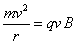(2)

Angular frequency of rotation is independent of velocity.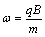(3)

An electron beam leaving the injector does not have a balance of radial forces. As discussed in reference , when electrons have no azimuthal velocity vo, the axial magnetic field exerts no radial force on the beam. The beam expands radially because of unbalanced spacecharge. The axial magnetic field will convert the radial velocity of electrons to azimuthal velocity resulting in focusing force. Ultimately, the beam results to its original radius and expansion begins anew. We look forward to calculate the value of total magnetic field and generation of formula. Thus, let us now consider the gyrofrequency of electrons.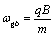(4)

←ωgb is known as gyro-frequency of electrons.

m is the mass of electrons

q is the charge on an electron

and B is the total magnetic field.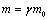(5)

mo = me = m = mass of electron

γ is known as relativistic factor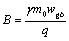(6)

B, the total magnetic field is thus related as above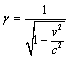(7)

v is known as beam velocity

c is the velocity of light in free space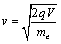(8)

V is applied voltage.

Next,

Let us put the value of v of (7) in B of (6) we get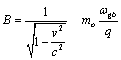(9)

Let us put the value of v from (8) in above expression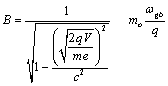(10)

Also,

ωgb = 2 π f

mo = me = mass of electron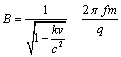(11)

where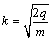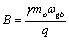(7) in (6)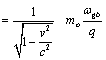(12)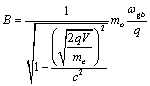(13)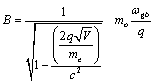ωgb = 2 π f

mo = me = mass of electron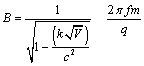(14)

where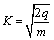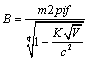(15)

Total magnetic field B is thus obtained.

Next, the magnetic field in axial direction is required.

As per , the magnetic field for either a wiggler or PPM configuration is of the following form:

B = ??Xm (16)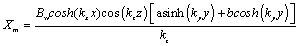(17)

If a=1 and b=0, a vertical field periodically varying with beam slightly moving back and forth horizontally is observed. If a=0 and b=1, the field is periodic permanent magnet (PPM) with axial field varying and beam rocking back and forth.

kx, ky and kz are known as wave numbers in x, y&z directions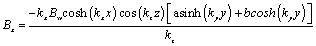(18)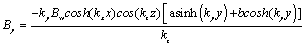(19)

Bz = Bω coshkx xcoskz z(asinhky y +bcoshky y)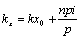(20)

p is known period of SWS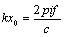(21)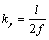(22)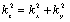(23)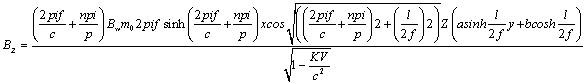(24)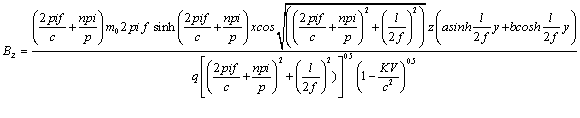(25)

above equation (25), gives relation of magnetic field with various parameters extensively involved in microwave generation like waveguide dimensions, specification of SWS and input parameters like voltage and operation frequency .

#### Results and Discussions

The relation has been validated theoretically and through simulation Zhaliang Wang et al. has taken an operational frequency of 36.6 GHz, SWS dimensions as 0.03 m,0.02 m, and 0.05 m, beam voltage as 160 Kev. A total magnetic field of 2.5 T(Tesla) & axial magnetic field of 1.3 T has been found experimentally. The same has been theoretically verified with the derived relation. The verification of the above results is done in following two ways

Theoretical validation

The experimental results, have been verified with the total applied magnetic field of 2.5 T and axial magnetic field is 1.37 T by standard 8 mm waveguide is taken . Input voltage of 160 KV sheet beam is taken operating frequency is 36.6 GHz. Dominant TM01 is considered. We can see good match in the values obtained experimentally and through the developed relation for calculation of axial magnetic field.

Verification through simulation

The pattern of axial magnetic field as per application is obtained from the developed relation. Figure 1 shows the axial magnetic field that can be generated through wiggler arrangement of permanent magnets. In the above diagram, the axial magnetic field propagates along z–direction, x and y the dimensions of waveguide. While in Figures 1-3, are the generalized cusp and permanent magnetic field respectively, generated through our equation, as we start to put specifications in the equation that we developed, we accordingly see more cusp kind of axial magnetic field as in Figure 4. Next is the simulation for the PPM which is the other kind of arrangements of magnets. In Figure 5 clearly shows a corrugationless, flat and disciplined beam when we put the specifications of our proposed model, in our developed magnetic field equation . Thus, a magnetic field relation involving various parameters of waveguide involved during generation of microwaves for magnetic field calculations is developed.

Figure 1: Simulation of axial magnetic field that propagates along z-direction, x and y are the dimensions of waveguide.

Figure 2: Simulation of axial magnetic field-more cusped in nature with increase in input voltage.

Figure 3: Simulation of axial magnetic field along z-direction-the flat field starts to appear.

Figure 4: Simulation of axial magnetic field along z-direction-the PPM field is seen.

Figure 5: Simulation of axial magnetic field along z-direction with 160kev input-corrugation less, flat, disciplined axial magnetic field is observed.

#### Conclusion

This with the derived expressions, exact value of axial magnetic field can be taken under vision for a desired frequency while planning experiments. The expression for axial magnetic field that extensively covers all parameters of waveguide is derived for the first time. The relation has been established to find the exact magnetic field for a desired operational frequency in terms of parameters like SWS dimensions, and beam voltage involved in the process of microwave generation.

#### References

Select your language of interest to view the total content in your interested language

### Article Usage

• Total views: 9462
• [From(publication date):
March-2016 - Jan 24, 2020]
• Breakdown by view type
• HTML page views : 9326Can't read the image? click here to refresh